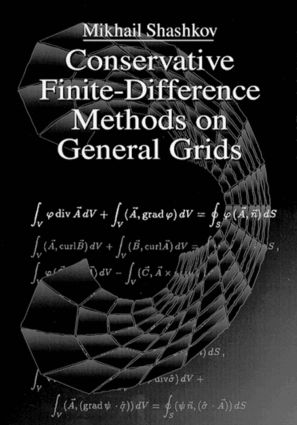Conservative Finite-Difference Methods on General Grids

1st Edition

CRC Press

384 pages

Purchasing Options:\$ = USD
Hardback: 9780849373756
pub: 1995-12-05
SAVE ~\$53.00
\$265.00
\$212.00
x
eBook (VitalSource) : 9781315140209
pub: 2018-02-06
from \$37.48

FREE Standard Shipping!

Description

This new book deals with the construction of finite-difference (FD) algorithms for three main types of equations: elliptic equations, heat equations, and gas dynamic equations in Lagrangian form. These methods can be applied to domains of arbitrary shapes. The construction of FD algorithms for all types of equations is done on the basis of the support-operators method (SOM). This method constructs the FD analogs of main invariant differential operators of first order such as the divergence, the gradient, and the curl. This book is unique because it is the first book not in Russian to present the support-operators ideas.

Conservative Finite-Difference Methods on General Grids is completely self-contained, presenting all the background material necessary for understanding. The book provides the tools needed by scientists and engineers to solve a wide range of practical engineering problems. An abundance of tables and graphs support and explain methods. The book details all algorithms needed for implementation. A 3.5" IBM compatible computer diskette with the main algorithms in FORTRAN accompanies text for easy use.

INTRODUCTION

Governing Equations

Elliptic Equations

Heat Equation

Equation of Gas Dynamic in Lagrangian Form

The Main Ideas of Finite-Difference Algorithms

1-D Case

2-D Case

Methods of Solution of Systems of Linear Algebraic Equation

Methods of Solution of Systems of Nonlinear Equations

METHOD OF SUPPORT-OPERATORS

Main Stages

The Elliptic Equations

Gas Dynamic Equations

System of Consistent Difference Operators in 1-D

Inner Product in Spaces of Difference Functions and Properties of Difference Operators

System of Consistent Difference Operators in 2-D

THE ELLIPTIC EQUATIONS

Introduction

Continuum Elliptic Problems with Dirichlet Boundary Conditions

Continuum Elliptic Problems with Robin Boundary Conditions

One-Dimensional Support Operator Algorithms

Nodal Discretization of Scalar Functions and Cell-Centered Discretization of Vector Functions

Cell-Valued Discretization of Scalar Functions and Nodal Discretization of Vector Functions

Numerical Solution of Test Problems

Two-Dimensional Support Operator Algorithms

Nodal Discretization of Scalar Functions and Cell-Valued Discretization of Vector Functions

Cell-Valued Discretization of Scalar Functions and Nodal Discretization of Vector Functions

Numerical Solution of Test Problems

Conclusion

Two-Dimensional Support Operator Algorithms

Discretization

Spaces of Discrete Functions

The Prime Operator

The Derived Operator

Multiplication by a Matrix and the Operator D

The Difference Scheme for the Elliptic Operator

The Matrix Problem

Approximation and Convergence Properties

HEAT EQUATION

Introduction

Finite-Difference Schemes for Heat Equation in 1-D

Finite-Difference Schemes for Heat Equation in 2-D

LAGRANGIAN GAS DYNAMICS

Kinematics of Fluid Motions

Integral Form of Gas Dynamics Equations

Integral Equations for One Dimensional Case

Differential Equations of Gas Dynamics in Lagrangian Form

The Differential Equations in 1D. Lagrange Mass Variables

The Statements of Gas Dynamics Problems in Lagrange Variables

Different Forms of Energy Equation

Acoustic Equations

Reference Information

Characteristic Form of Gas Dynamics Equations

Riemann's Invariants

Discontinuous Solutions

Conservation Laws and Properties of First Order Invariant Operators

Finite-Difference Algorithm in 1D

Discretization in 1D

Discrete Operators in 1D

Semi-Discrete Finite-Difference Scheme in 1D

Fully Discrete, Explicit, Computational Algorithm

Computational Algorithm-New Time Step-Explicit Finite-Difference Scheme

Computational Algorithm-New Time Step-Implicit Finite-Difference Scheme

Stability Conditions

Homogeneous Finite-Difference Schemes. Artificial Viscosity

Artificial Viscosity in 1D

Numerical Example

Finite Difference Algorithm in 2D

Discretization in 2D

Discrete Operators in 2D

Semi-Discrete Finite-Difference Scheme in 2D

Stability Conditions

Finite-Difference Algorithm in 2D

Computational Algorithm-New Time Step-Explicit Finite-Difference Scheme

Computational Algorithm-New Time Step-Implicit Finite-Difference Scheme

Artificial Viscosity in 2D

Numerical Example

APPENDIX: FORTRAN CODE DIRECTORY

General Description of Structure of Directories on the Disk

Programs for Elliptic Equations

Programs for 1D Equations

Programs for 2D Equations

Programs for Heat Equations

Programs for 1D Equations

Programs for 2D Equations

Programs for Gas Dynamics Equations

Programs for 1D Equations

Programs for 2D Equations

Bibliography

Shashkov\, Mikhail